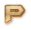# Python Bitwise Online Test

Instructions:
• Total number of questions : 10.
• Time alloted : 10 minutes.
• Each question carry 1 mark.
• No Negative marks
• DO NOT refresh the page.
• All the best :-)

### What You Will Learn in

This Python Bitwise Online Test helps you to Boost your Knowledge in Python. In this test, we will cover the topics in the form of questions like

• The binary form of 1 is 0001.
• The symbol '&' represents bitwise AND.
• The ^ operator represent bitwise XOR operation.
• The prefix 0x specifies that the value is hexadecimal in nature.
• << is the bitwise left shift operator.
• bin() function converts the decimal into binary.